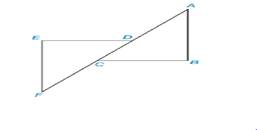Chapter 3.2, Problem 20E### Elementary Geometry for College St...

6th Edition
Daniel C. Alexander + 1 other
ISBN: 9781285195698

#### Solutions

Chapter
Section### Elementary Geometry for College St...

6th Edition
Daniel C. Alexander + 1 other
ISBN: 9781285195698
Textbook Problem
23 views

# In Exercise 17 to 20, first prove that triangles are congruent then use CPCTC. Given: D E ¯ ⊥ E F ¯ and C B ¯ ⊥ A B ¯ A B ¯ ∥ F E ¯ A C ¯ ≅ F D ¯Prove: E F ¯ ≅ B A ¯

To determine

To prove:

The given statement.

Explanation

Given:

The following figure shows the given diagram.

Given that,

DE¯EF¯ and CB¯AB¯

AB¯FE¯

AC¯FD¯

Approach:

By definition of ASA, if two angles and their included side of one triangle is congruent to two angles and their included side of second triangle, then triangle will be congruent.

The completed proof is shown in the following table.

 PROOF Statements Reasons 1. DE¯⊥EF¯ and CB¯⊥AB¯ 1. Given 2. ∠B≅∠E 2. The angles formed by perpendicular lines are right angles and right angles are congruent.. 3. AB¯∥FE¯ 3. Given 4. ∠F≅∠A 4. If two parallel lines are cut by a transversal, then the alternate interior angles will be congruent. 5. AC¯≅FD¯ 5. Given 7

### Still sussing out bartleby?

Check out a sample textbook solution.

See a sample solution

#### The Solution to Your Study Problems

Bartleby provides explanations to thousands of textbook problems written by our experts, many with advanced degrees!

Get Started

#### 165/8161/2167/8

Applied Calculus for the Managerial, Life, and Social Sciences: A Brief Approach

#### Evaluate the integral. 18. ecos2d

Single Variable Calculus: Early Transcendentals

#### For j – 2k 3i – 4k 3i + j – 2k 0 (zero vector)

Study Guide for Stewart's Multivariable Calculus, 8th

#### Graph each function. fx=logx-2

College Algebra (MindTap Course List)